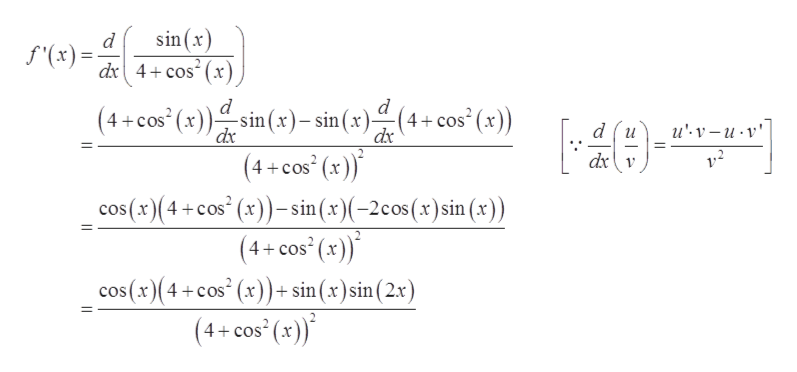Question
10 views

Consider the function on the interval (0, 2π).

Find the open intervals on which the function is increasing or decreasing. (Enter your answers using interval notation.)

increasing :

decreasing :

Apply the First Derivative Test to identify the relative extrema.

relative maximum :

relative minimum :

check_circle

Step 1

Given, function is

Step 2

For critical points of function, differentiate function and equal to zero.

Differentiate equation (1) with respect to ‘x’,help_outlineImage Transcriptionclosesin (x) d (x)= dx4cos(x d )(4+cos'(x)) (4+cos (x)sin (x)- sin(x) (4+cos" (x)) _ du u'v-и-v" dx dx dxv cos(x)(4+cos (x))-sin (x)(-2cos(x)sin (x)) (4+cos (x)) cos(x)(4 cos (x))+ sin(x)sin(2x) 4+cos2 (x) fullscreen
Step 3

Take derivative is e...

### Want to see the full answer?

See Solution

#### Want to see this answer and more?

Solutions are written by subject experts who are available 24/7. Questions are typically answered within 1 hour.*

See Solution
*Response times may vary by subject and question.
Tagged in

### Derivative